# Introduction

vtree is a flexible tool for calculating and displaying variable trees — diagrams that show information about nested subsets of a data frame. vtree can be used to:

1. explore a data set interactively

2. produce customized figures for reports and publications.

Note, however, that vtree is not designed to build or display decision trees.

Given a data frame and simple specifications, vtree will produce a variable tree and automatically label it with counts, percentages, and other summaries.

The sections below introduce variable trees and provide an overview of the features of vtree. Or you can skip ahead and start using the `vtree` function.

## Two examples

Subsets play an important role in almost any data analysis. Imagine a data set of countries that includes variables named `population`, `continent`, and `landlocked`. Suppose we wish to examine subsets of the data set based on the `continent` variable. Within each of these subsets, we could examine nested subsets based on the `population` variable, for example, countries with populations under 30 million and over 30 million. We might continue to a third nesting based on the `landlocked` variable.

Nested subsets are at the heart of questions like the following: Among African countries with a population over 30 million, what percentage are landlocked? The variable tree below provides the answer: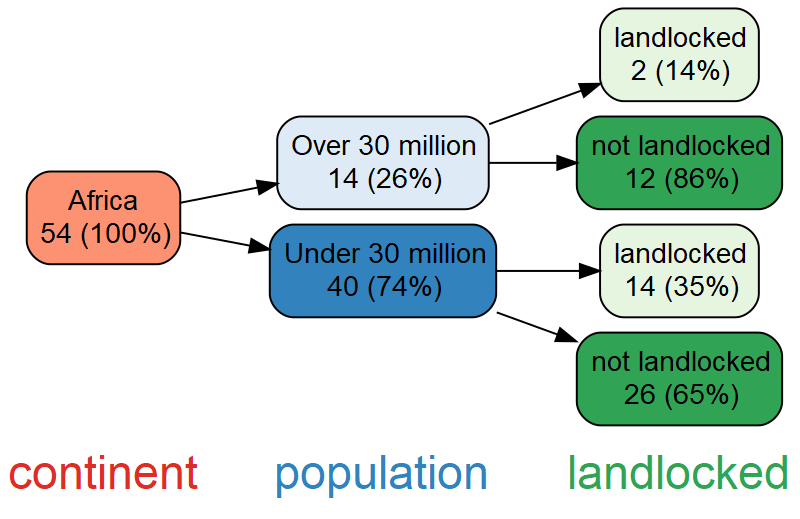Even in simple situations like this, it can be a chore to keep track of nested subsets and calculate the corresponding percentages. The denominator used to calculate percentages may also depend on whether the variables have any missing values, as discussed later. Finally, as the number of variables increases, the magnitude of the task balloons, because the number of nested subsets grows exponentially. vtree provides a general solution to the problem of calculating nested subsets and displaying information about them.

Nested subsets arise in all kinds of situations. Consider, for example, flow diagrams for clinical studies, such as the following CONSORT-style diagram, produced by vtree.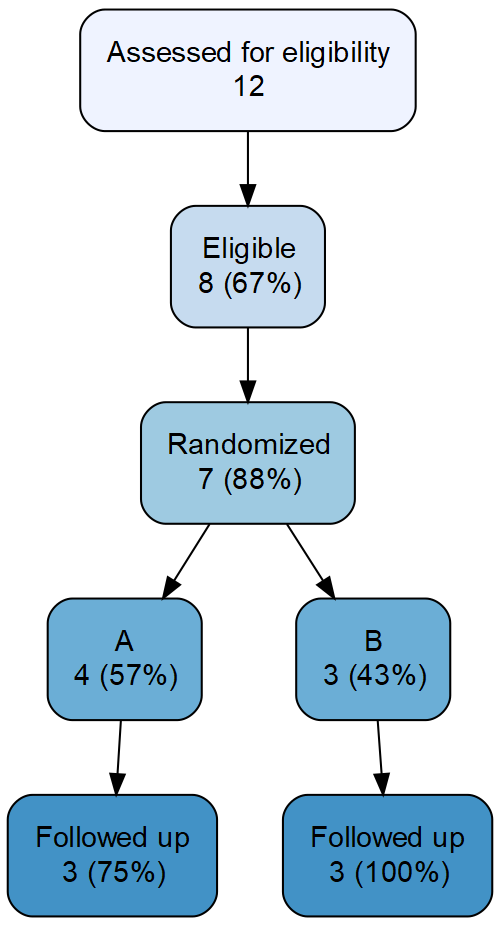Both the structure of this variable tree and the numbers shown were automatically determined. When manual calculation and transcription are instead used to populate diagrams like this, mistakes are likely. And although the errors that make it into published articles are often minor, they can sometimes be disastrous. One motivation for developing vtree was to make flow diagrams reproducible. The ability to reproducibly generate variable trees also means that when a data set is updated, a revised tree can be automatically produced.

At the end of this vignette, there is a collection of examples of variable trees using R datasets that you can try.

## Basic features of a variable tree

The examples that follow use a data set called `FakeData` which represents 46 fictitious patients. We’ll start by using just two variables, although variable trees are especially useful with three or more variables. The variable tree below depicts subsets defined by `Sex` (M or F) nested within subsets defined by disease `Severity` (Mild, Moderate, Severe, or NA).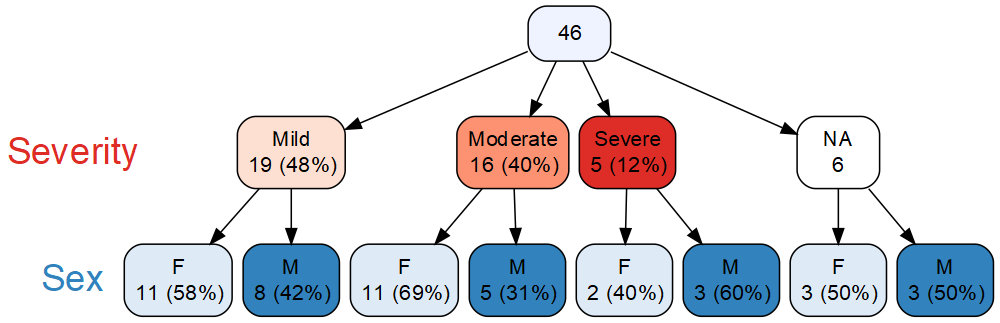A variable tree consists of nodes connected by arrows. At the top of the diagram above, the root node of the tree contains all 46 patients. The rest of the nodes are arranged in successive layers, where each layer corresponds to a specific variable. Note that this highlights one difference between variable trees and some other kinds of trees: each layer of a variable tree corresponds to just one variable. (In a decision tree, by contrast, different branches can have different sequences of variable splits.)

Continuing with the variable tree above, the nodes immediately below the root represent values of `Severity` and are referred to as the children of the root node. In this case, `Severity` was missing (NA) for 6 patients, and there is a node for these patients. Inside each of the nodes, the number of patients is displayed and—except for in the missing value node—the corresponding percentage is also shown. Note that, by default, `vtree` displays “valid” percentages, i.e. the denominator used to calculate the percentage is the total number of non-missing values, 40.

The final layer of the tree corresponds to values of `Sex`. These nodes represent males and females within subsets defined by each value of `Severity`. In each of these nodes the percentage is calculated in terms of the number of patients in its parent node.

Like any node, a missing-value node can have children. For example, of the 6 patients for whom `Severity` is missing, 3 are female and 3 are male. By default, `vtree` displays the full missing-value structure of the specified variables.

Also by default, `vtree` automatically assigns a color palette to the nodes of each variable. `Severity` has been assigned red hues (lightest for Mild, darkest for Severe), while `Sex` has been assigned blue hues (light blue for females, dark blue for males). The node representing missing values of `Severity` is colored white to draw attention to it.

## Variable trees compared to contingency tables

A tree with two variables is similar to a two-way contingency table. In the example above, `Sex` is shown within levels of `Severity`. This corresponds to the following contingency table, where the percentages within each column add to 100%. These are called column percentages.

Mild Moderate Severe NA
F 11 (58%) 11 (69%) 2 (40%) 3 (50%)
M 8 (42%) 5 (31%) 3 (60%) 3 (50%)

Likewise, a tree with `Severity` shown within levels of `Sex` corresponds to a contingency table with row percentages.

While the contingency table above is more compact than the corresponding variable tree, some people find the variable tree more intuitive. When three or more variables are of interest, multi-way contingency tables are often used. These are typically displayed using several two-way tables, but as the number of variables increases, these become increasingly difficult to interpret. Variable trees, on the other hand, have the same simple structure regardless of the number of variables.

Note that contingency tables are not always more compact than variable trees. When most cells of a large contingency table are empty (in which case the table is said to be sparse), the corresponding variable tree may be more compact since empty nodes are not shown.

## Features of vtree

vtree is designed to be quick and easy to use, so that it is convenient for data exploration, but also flexible enough that it can be used to prepare publication-ready figures. To generate a basic variable tree, it is only necessary to specify a data frame and some variable names. However extra features extend this basic functionality to provide:

In many cases, you may wish to generate several different variable trees to investigate a collection of variables in a data frame. For example, it is often useful to change the order of variables, prune parts of the tree, etc.

## Technical overview

vtree is built on open-source software: in particular Richard Iannone’s DiagrammeR package, which provides an interface to the Graphviz software using the htmlwidgets framework. Additionally, vtree makes use of the Shiny package, and the svg-pan-zoom JavaScript library.

A formal description of variable trees follows.

The root node of the variable tree represents the entire data frame. The root node has a child for each observed value of the first variable that was specified. Each of these child nodes represents a subset of the data frame with a specific value of the variable, and is labeled with the number of observations in the subset and the corresponding percentage of the number of observations in the entire data frame. The nth layer below the root of the variable tree corresponds to the nth variable specified. Apart from the root node, each node in the variable tree represents the subset of its parent defined by a specific observed value of the variable at that layer of the tree, and is labeled with the number of observations in that subset and the corresponding percentage of the number of observations in its parent node.

Note that a node always represents at least one observation. And unlike a contingency table, which can have empty cells, a variable tree has no empty nodes.

# The `vtree` function

Consider a data frame named `df`, which includes discrete variables `v1` and `v2`. Suppose we wish to produce a variable tree showing subsets based on values of `v1` as well as subsets of those subsets based on values of `v2`. The variable tree can be displayed using the following command:

``vtree(df,"v1 v2")``

Alternatively, you may wish to assign the output of `vtree` to an object:

``simple_tree <- vtree(df,"v1 v2")``

Then it can be displayed later using:

``simple_tree``

Suppose `vtree` is called without a list of variables:

``vtree(df)``

In this case, only the root node is shown, representing the entire data frame. Although a tree with just one node might not seem very useful, we’ll see later that summary information about the whole data frame can be displayed there.

The `vtree` function has numerous optional parameters. For example, by default `vtree` produces a horizontal tree (that is, a tree that grows from left to right). To generate a vertical tree, specify `horiz=FALSE`.

## Mini tutorial

This section introduces some basic features of the `vtree` function.

To display a variable tree for a single variable, say `Severity`, use the following command:

``vtree(FakeData,"Severity")``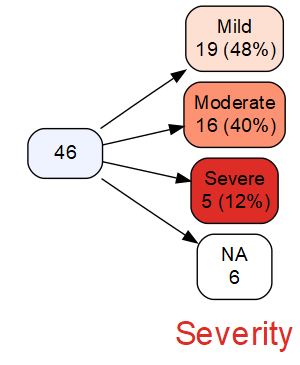By default, next to each layer of the tree, a variable name is shown. In the example above, “Severity” is shown below the corresponding nodes. (For a vertical tree, “Severity” would be shown to the left of the nodes.) If you specify `showvarnames=FALSE`, no variable names will be shown.

`vtree` can also be used with dplyr. For example, to rename the `Severity` variable as `HowBad`, we can pipe the data frame into the `rename` function in dplyr, and then pipe the result into `vtree`:

``````library(dplyr)

Note that `vtree` also has a built-in way of renaming variables, which is an alternative to using dplyr.

Large variable trees can be difficult to display in a readable way. One approach that helps is to display the count and percentage on the same line in each node. For example, in the tree above, the label for the Moderate node is on two lines, like this:

Moderate
16 (40%)

Specifying `sameline=TRUE` results in single-line labels, like this:

Moderate, 16 (40%)

### Percentages

By default, vtree shows “valid percentages”, i.e. percentages calculated using the total number of non-missing values as denominator. In the case of `Severity`, there are 6 missing values, so the denominator is 46 - 6, or 40. There are 19 Mild cases, and 19/40 = 0.475 so the percentage shown is 48%. No percentage is shown in the NA node since missing values are not included in the denominator.

If you prefer the denominator to represent the complete set of observations (including any missing values), specify `vp=FALSE`. A percentage will be shown in each of the nodes, including any NA nodes.

If you don’t wish to see percentages, specify `showpct=FALSE`, and if you don’t wish to see counts, specify `showcount=FALSE`.

### Displaying a legend and hiding node labels

To display a legend, specify `showlegend=TRUE`. Next to each variable name are “legend nodes” representing the values of that variable and colored accordingly. For each variable, the legend nodes are grouped within a light gray box. Each legend node also contains a count (with a percentage) for the value represented by that node in the whole data frame. This is known as the marginal count (and percentage).

When the legend is shown, labels in the nodes of the variable tree are redundant, since the colors of the nodes identify the values of the variables (although the labels may aid readability). If you prefer, you can hide the node labels, by specifying `shownodelabels=FALSE`:

``vtree(FakeData,"Severity Sex",showlegend=TRUE,shownodelabels=FALSE)``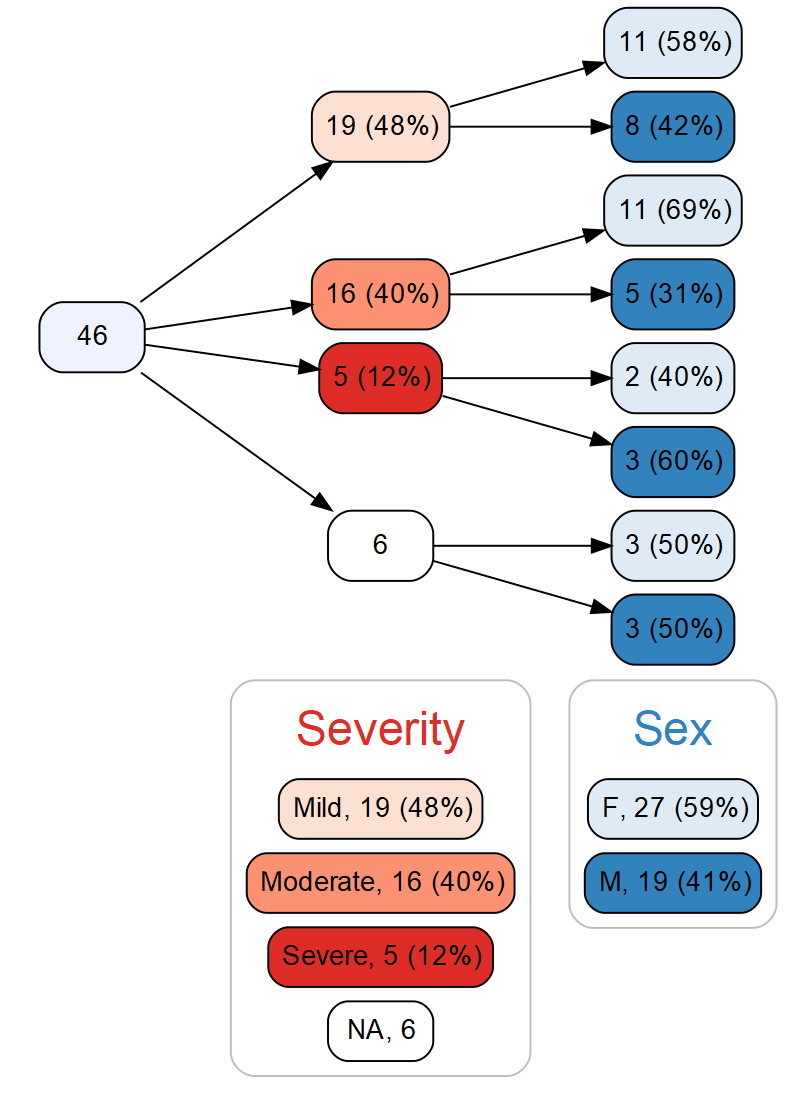Since `Severity` is the first variable in the tree, it is not nested within another variable. Therefore the marginal counts and percentages for `Severity` shown in the legend nodes are identical to those displayed in the nodes of the variable tree. In contrast, for `Sex`, the marginal counts and percentages are different from what is shown in the nodes of the variable tree for `Sex` since they are nested within levels of `Severity`.

### Text wrapping

By default, `vtree` wraps text onto the next line whenever a space occurs after at least 20 characters. This can be adjusted, for example, to 15 characters, by specifying `splitwidth=15`. To disable line splitting, specify `splitwidth=Inf` (`Inf` means infinity, i.e. “do not split”.)

The `vsplitwidth` parameter is similarly used to control text wrapping in variable names. This is helpful with long variable names, which may be truncated unless wrapping is used. In this case text wrapping occurs not only at spaces, but also at any of the following characters:

``. - + _ = / (``

For example if `vsplitwidth=5`, a variable name like `First_Emergency_Visit` would be split into

`First_`
`Emergency_`
`Visit`

This concludes the mini-tutorial. vtree has many more features, described in the following sections.

## Pruning

This section shows how to remove branches from a variable tree.

When a variable tree gets too big, or you are only interested in certain parts of the tree, it may be useful to remove some nodes along with their descendants. This is known as pruning. For convenience, there are several different ways to prune a tree, described below.

### The `prune` parameter

Here’s a variable tree we’ve already seen in various forms:

``vtree(FakeData,"Severity Sex")``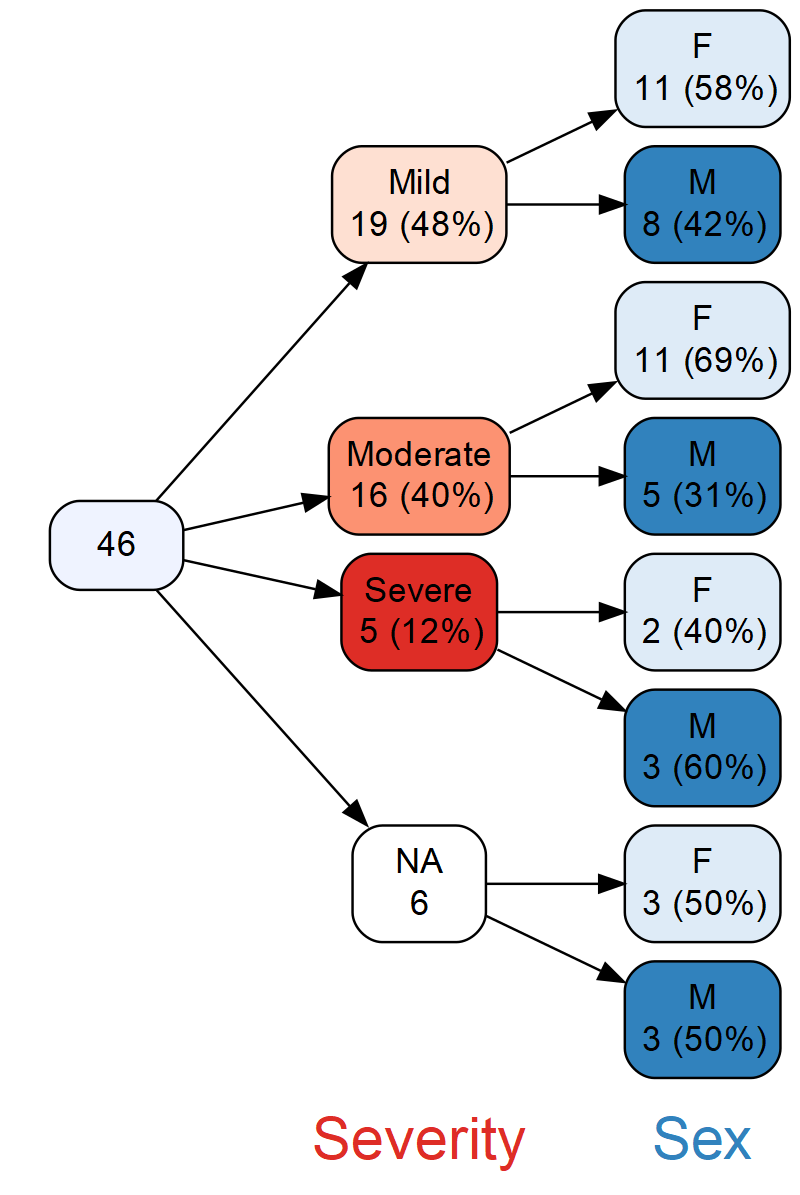Suppose you don’t want the tree to show branches for individuals whose disease is Mild or Moderate. Specifying `prune=list(Severity=c("Mild","Moderate"))` removes those nodes, and all of their descendants:

``vtree(FakeData,"Severity Sex",prune=list(Severity=c("Mild","Moderate")))``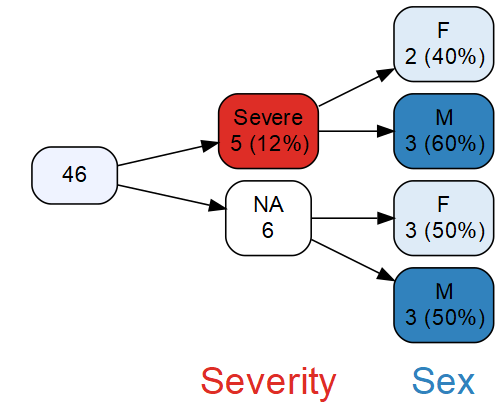In general, the argument of the `prune` parameter is a list with an element named for each variable you wish to prune. In the example above, the list has a single element, named `Severity`. In turn, that element is a vector `c("Mild","Moderate")` indicating the values of `Severity` to prune.

Caution: Once a variable tree has been pruned, it is no longer complete. This can sometimes be confusing since not all observations are represented at certain layers of the tree. For example in the tree above, only 11 observations are shown in the `Severity` nodes and their children.

### The `keep` parameter

Sometimes it is more convenient to specify which nodes should be retained rather than which ones should be discarded. The `keep` parameter is used for this purpose, and can thus be considered the complement of the `prune` parameter. For example, to retain the Moderate `Severity` node:

``vtree(FakeData,"Severity Sex",keep=list(Severity="Moderate"))``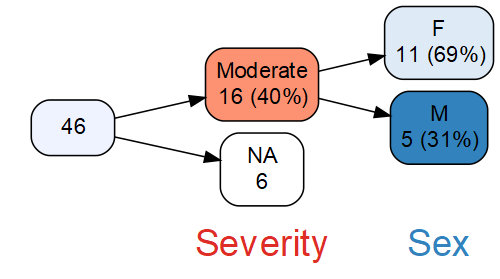Note: In addition to the Moderate node, the missing value node has also been retained. In general, whenever valid percentages are used (which is the default), missing value nodes are retained when `keep` is used. This is because valid percentages are difficult to interpret without knowing the denominator, which requires knowing the number of missing values.

On the other hand, here’s what happens when `vp=FALSE`:

``vtree(FakeData,"Severity Sex",keep=list(Severity="Moderate"),vp=FALSE)``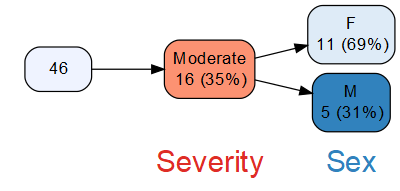### The `prunebelow` parameter

As seen above, a disadvantage of pruning is that in the resulting tree, the counts shown in child nodes may not add up to the counts shown in their parent node.

An alternative is to prune below the specified nodes (i.e. to prune their descendants), so that the counts always add up. In the present example, this means that the Mild and Moderate nodes will be shown, but not their descendants. The `prunebelow` parameter is used to do this:

``vtree(FakeData,"Severity Sex",prunebelow=list(Severity=c("Mild","Moderate")))``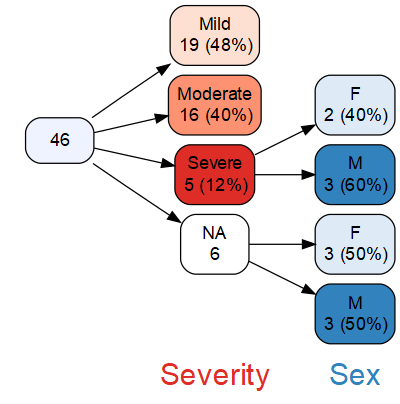### The `follow` parameter

The complement of the `prunebelow` function is the `follow` function. Instead of specifying which nodes should be pruned below, this allows you to specify which nodes should be “followed” (that is, not pruned below).

### The `prunesmaller` parameter

As a variable tree grows, it can become difficult to see the forest for the tree. For example, the following tree is hard to read, even when `sameline=TRUE` has been specified:

``vtree(FakeData,"Severity Sex Age Category",sameline=TRUE)``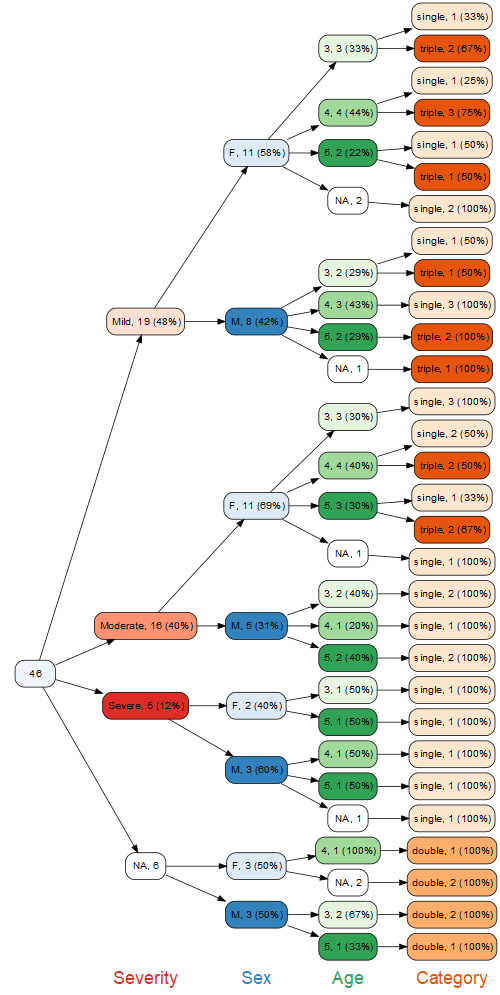One solution is to prune nodes that contain small numbers of observations. For example if you want to only see nodes with at least 3 observations, you can specify `prunesmaller=3`, as in this example:

``vtree(FakeData,"Severity Sex Age Category",sameline=TRUE,prunesmaller=3)``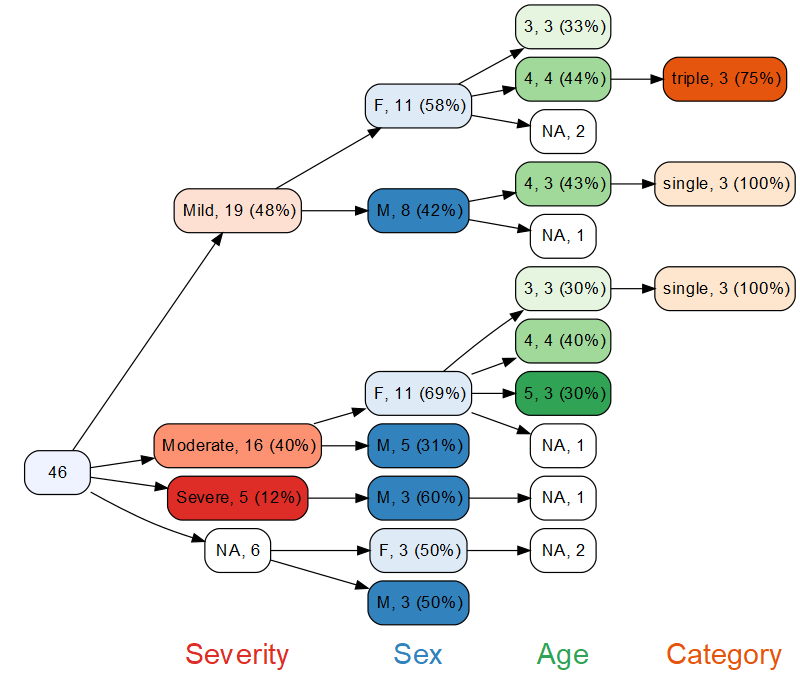As with the `keep` parameter, when valid percentages are used (`vp=TRUE`, which is the default), nodes represent missing values will not be pruned. (As noted previously, this is because percentages are confusing when missing values are not shown.) On the other hand, when `vp=FALSE`, missing nodes will be pruned (if they are small enough).

## Labels for variables and nodes

This section shows how to relabel variables and nodes.

By default, `vtree` labels variables and nodes exactly as they appear in the data frame. But it is often useful to change these labels.

### Changing variable labels with the `labelvar` parameter

Suppose `Severity` in fact represents initial severity. To label it that way in the variable tree, specify `labelvar=c(Severity="Initial severity")`:

``vtree(FakeData,"Severity Sex",horiz=FALSE,labelvar=c(Severity="Initial severity"))``